### Sample Paper Set 3

Sample Paper Set 3### Class 9 - Maths - Sample Paper 2019 - Set 2

Class 9 - Maths - Sample Paper 2019 - Set 2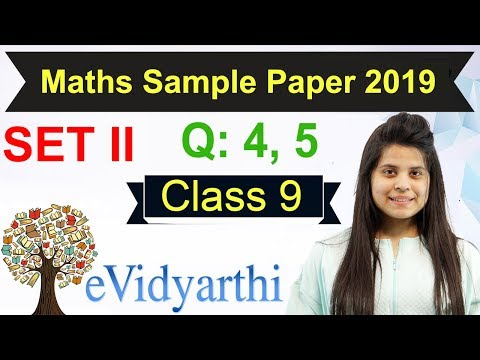### Linear Equations In Two Variables - R.D Sharma Cla

Linear Equations In Two Variables - R.D Sharma Cla### Class 9 Maths Sample Paper 2019

Class 9 Maths Sample Paper 2019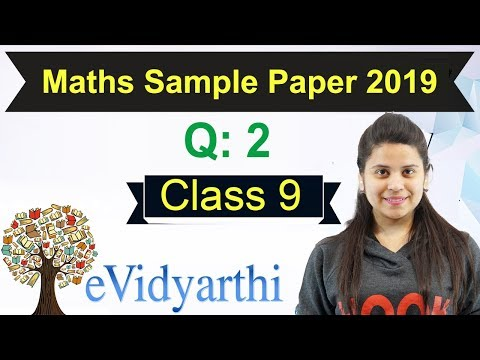### Statistics - R.D Sharma Class 9 Maths - Chapter 14

Statistics - R.D Sharma Class 9 Maths - Chapter 14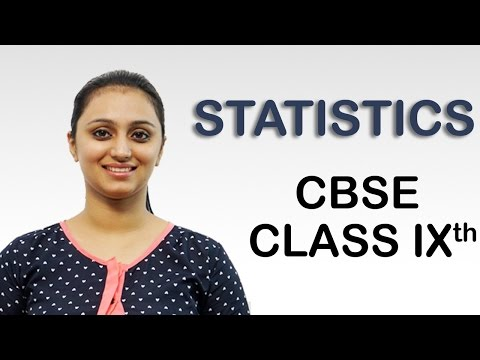### Probability - R D Sharma Class 9 Maths - Chapter 1

Probability - R D Sharma Class 9 Maths - Chapter 1### Areas of Parallelograms and Triangles - Class 9 M

Areas of Parallelograms and Triangles - Class 9 M### Coordinate Geometry, Class 9 R D Sharma Maths, Cha

Coordinate Geometry, Class 9 R D Sharma Maths, Cha### Circles - R.D Sharma Class 9 Maths - Chapter 10

Circles - R.D Sharma Class 9 Maths - Chapter 10### Surface Areas & Volumes - R.D Sharma Class 9 Maths

Surface Areas & Volumes - R.D Sharma Class 9 Maths### Lines And Angles, R D Sharma Class 9 Maths, Chapte

Lines And Angles, R D Sharma Class 9 Maths, Chapte### Quadrilaterals - R D Sharma Class 9 Maths - Chapte

Quadrilaterals - R D Sharma Class 9 Maths - Chapte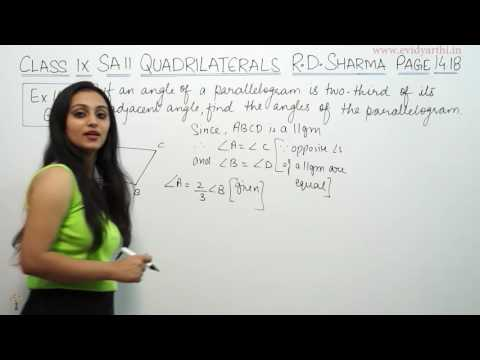### Euclid’s Geometry - Class 9 Maths Solution R D Sha

Euclid’s Geometry - Class 9 Maths Solution R D Sha### Polynomials - R. D Sharma Class 9 Maths - Chapter

Polynomials - R. D Sharma Class 9 Maths - Chapter### Number Systems, Class 9 R D Sharma Maths Chapter 1

Number Systems, Class 9 R D Sharma Maths Chapter 1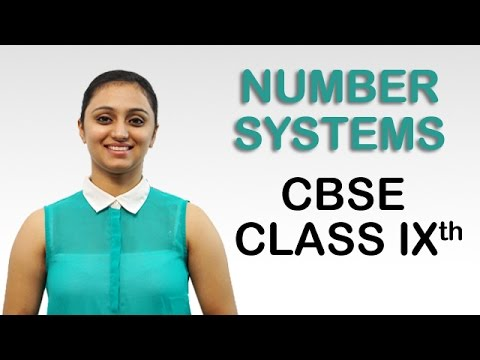### Triangles - R. D. Sharma Class 9 Maths - Chapter 7

Triangles - R. D. Sharma Class 9 Maths - Chapter 7### Triangles - Class 9 Maths - Chapter 7

Triangles - Class 9 Maths - Chapter 7### Heron Formula - Class 9 Maths - Chapter 12

Heron Formula - Class 9 Maths - Chapter 12### Statistics - Class 9 Maths - Chapter 14

Statistics - Class 9 Maths - Chapter 14### Areas of Parallelograms and Triangles - Class 9 Ma

Areas of Parallelograms and Triangles - Class 9 Ma### Coordinate Geometry - Class 9 Maths - Chapter 3

Coordinate Geometry - Class 9 Maths - Chapter 3### Surface Areas & Volumes - Class 9 Maths - Chapter

Surface Areas & Volumes - Class 9 Maths - Chapter### Circles - Class 9 Maths - Chapter 10

Circles - Class 9 Maths - Chapter 10### Lines And Angles - Class 9 Maths - Chapter 6

Lines And Angles - Class 9 Maths - Chapter 6### Quadrilaterals - Class 9 Mathematics - Chapter 8

Quadrilaterals - Class 9 Mathematics - Chapter 8### Linear Equations In Two Variables - Class 9 Maths

Linear Equations In Two Variables - Class 9 Maths### Euclid’s Geometry - Class 9 Maths - Chapter 5

Euclid’s Geometry - Class 9 Maths - Chapter 5### Polynomials - Class 9 Maths - Chapter 2

Polynomials - Class 9 Maths - Chapter 2### Probability - CBSE Class 9 Mathematics

Probability - CBSE Class 9 Mathematics### Number Systems - CBSE Class 9 Mathematics

Number Systems - CBSE Class 9 Mathematics